# AP Calculus AB Practice Test 46

### Test Information10 questions20 minutes

Calculator Disallowed

1.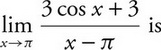2.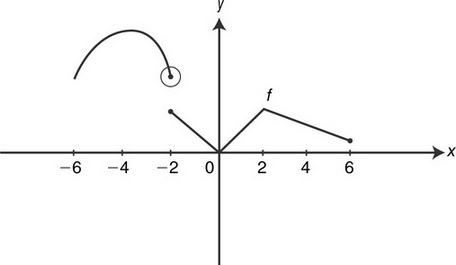The figure above shows the graph of a piecewise function. The graph has a horizontal tangent at x= -4. What are all the values of x, such that -6 < x< 6, at which fis continuous but not differentiable?

3.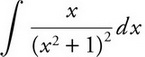4.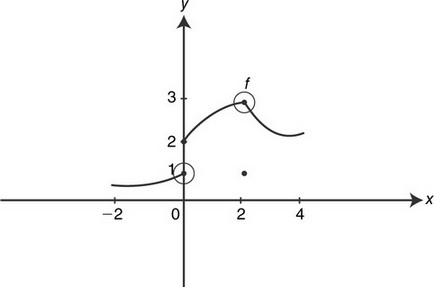The diagram above shows the graph of a function ffor -2 ≤ x≤4. Which of the following statements is/are true?

I.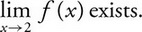II.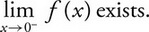III.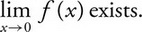5.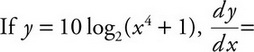6.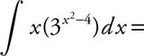7.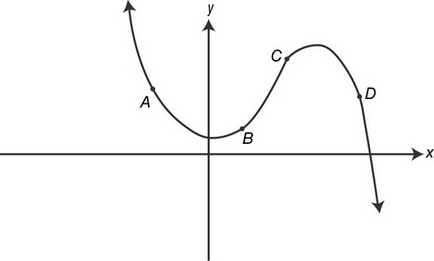In the figure above, which of the four given points on the graph is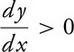and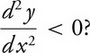8.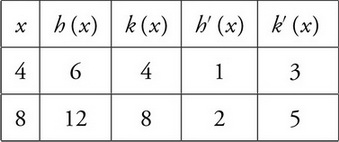The table above shows the values of the functions hand kand their derivatives at x= 4 and x= 8. What is the value of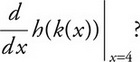9. If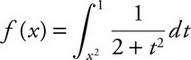, then f′(2)=

10.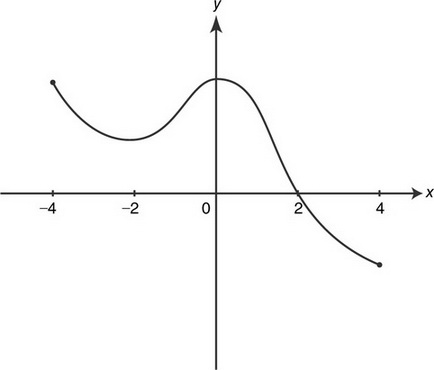The figure above shows the graph of a continuous function ffor -4 ≤ x≤ 4. On what intervals is f′(x) > 0 for all values ofx?